## Example Questions

### Example Question #3 : How To Simplify A Fraction

Simplify the given fraction: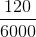Possible Answers: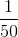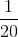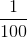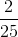Correct answer:Explanation:

120 goes into 6000 evenly 50 times, so we get 1/50 as our simplified fraction.

### Example Question #33 : Algebraic Fractions

Simplify the given fraction: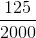Possible Answers: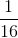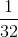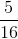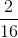Correct answer:Explanation:

125 goes into 2000 evenly 16 times. 1/16 is the fraction in its simplest form.

### Example Question #21 : How To Simplify A Fraction

Simplify the following expression: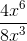Possible Answers: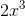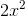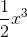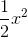Correct answer:Explanation:

Following this equation, you divide 4 by 8 to get 1/2. When a variable is raised to an exponent, and you are dividing, you subtract the exponents, so 6 – 3 = 3.

### Example Question #11 : Algebraic Fractions

Reduce the fraction: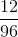Possible Answers: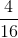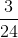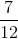Correct answer:Explanation:

The numerator and denominator are both divisible by 12. Thus, we divide both by 12 to get our final answer.

If we instead divide by another common factor, we may need to complete the process again to make sure that we have completely reduced the fraction.

In mathematical words we get the following: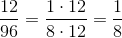### Example Question #11 : Algebraic Fractions

Simplify: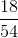Possible Answers: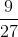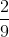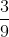Correct answer:Explanation:is one third of, soin its simplest form is. To reach this answer, divide bothandby.

### Example Question #12 : Algebraic Fractions

What is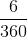in its simplest form?

Possible Answers: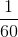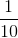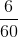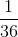Correct answer:Explanation:

To reach the answer, divide both the numerator and denominator by. After doing that, the answer is.

### Example Question #2521 : Act Math

Simplify: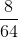Possible Answers: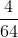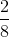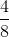Correct answer:Explanation:

To find the answer, divide both the numerator and the denominator by. The result is.

### Example Question #1 : How To Evaluate A Fraction

Evaluate the following equation whenand round your answer to the nearest hundredth.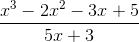Possible Answers: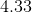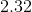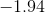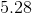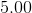Correct answer:Explanation:1. Plug inwherever there is anin the above equation.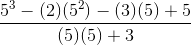2. Perform the above operations.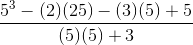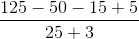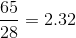### Example Question #1 : How To Evaluate A Fraction

Mary walked to school at an average speed of 2 miles per hour and jogged back along the same route at an average speed of 6 miles per hour. If her total traveling time was 1 hour, what was the total number of miles in the round trip?

Possible Answers:Correct answer:Explanation:

Since Mary traveled 3 times as quickly coming from school as she did going to school (6 miles per hour compared to 2 miles per hour), we know that Mary spent only a third of the time coming from school as she did going. If x represents the number of hours it took to get to school, then x/3 represents the number of hours it took her to return.

Knowing that the total trip took 1 hour, we have:

x/3 = 1

3x/3 + 1x/3 = 1

4x/3 = 1

= 3/4

So we know it took Mary 3/4 of an hour to travel to school (and the remaining 1/4 of an hour to get back).

Remembering that distance =  rate * time, the distance Mary traveled on her way to school was (2 miles per hour) * (3/4 of an hour) = 3/2 miles. Furthermore, since she took the same route coming back, she must have traveled 3/2 of a mile to return as well.

Therefore, the the total number of miles in Mary's round trip is 3/2 miles + 3/2 miles = 6/2 miles = 3 miles.

### Example Question #3 : How To Evaluate A Fraction

If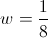then which of the following is equal to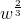?

Possible Answers: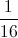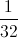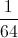Correct answer:Explanation:

To raise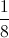to the exponent, squareand then take the cube root.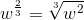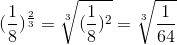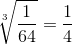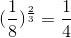### All ACT Math Resources